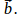## Notation

Tensors are a generalisation of vectors. A vector may be written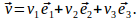The same vector can be written in tensor notation as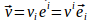where subscripts and superscripts in the same expression indication summation.
A tensor may have any number of superscripts and subscripts, with each element of a subscript or superscript taking a range of possible values.
If there is one subscript or superscript it is called first order.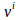and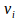are first order.
If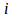can take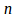possible values then v has n components.
If the number of subscript or superscript elements add to two, the system is second order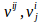and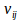are all second order.
Ifcan takepossible values then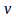has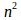components.
If the number of subscript or superscript elements add to three, the system is third order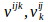and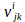and
$\mathbf{v}_{ijk}$
are all third order.
Ifcan takepossible values thenhas
$n^3$
components.
The dot product of vectors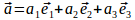and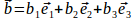is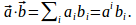If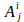is a matrix with entry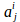in the ith row, jth column, then we can find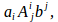called the generaled dot product of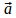and